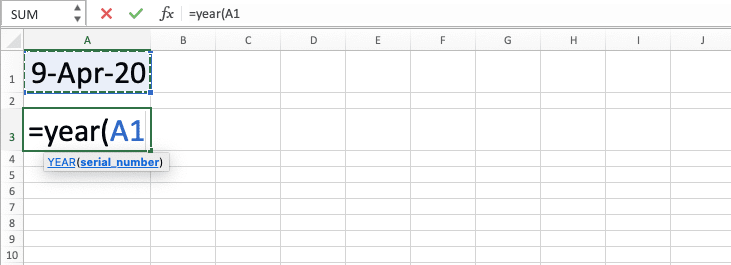How to Use the YEAR Formula in Excel: Functions, Examples, and Writing Steps - Compute Expert

# How to Use the YEAR Formula in Excel: Functions, Examples, and Writing Steps

Home >> Excel Tutorials from Compute Expert >> Excel Formulas List >> How to Use the YEAR Formula in Excel: Functions, Examples, and Writing Steps

In this tutorial, you will learn how to use the YEAR formula in excel completely.

When working with dates in excel, we may sometimes need to get the year number of our dates. We can get that number easily if we use the YEAR formula.

Disclaimer: This post may contain affiliate links from which we earn commission from qualifying purchases/actions at no additional cost for you. Learn more

## What is the YEAR Formula in Excel?

YEAR formula in excel is a formula that helps you to extract the year number of a date in excel.

## YEAR Function in Excel

You can use YEAR to get a date’s year number in excel.

## YEAR Result

The YEAR result is a number that represents the year of the date you input into this formula.

## Excel Version from Which We Can Start Using YEAR

We can start using YEAR in excel since excel 2003.

## The Way to Write It and Its Input

Here is the general writing form of YEAR in excel

= YEAR ( date )

You only need to input the date you want to get the year number from to YEAR.

You can also input a serial number that represents the date in excel if you want. The number is 1 to 2,958,465, which is equal to 1 January 1900 to 31 December 9999.

## Example of Its Usage and Result

Here is an implementation example of the YEAR formula in excel.As you can see there, it is easy to use YEAR to extract a year number from our date in excel. Just input the date you want to extract the year number from to the YEAR formula you write.

Press enter after you write the formula and you will immediately get your date’s year number!

## Writing Steps

After we discussed the YEAR writing form, input, and implementation example, now let’s discuss its writing steps. As YEAR only needs your date input to function, it is pretty easy to follow its writing steps.

1. Type an equal sign ( = ) in the cell where you want to put your date’s year number2. Type YEAR (can be with large and small letters) and an open bracket sign after =3. Input the date you want to get the year number from or the cell coordinate where that date is4. Type a close bracket sign5. Press Enter
6. Done!## How to Determine if a Date is in a Leap Year or Not: IF MOD YEAR

Want to know whether your date is in a leap year or not? You can combine the IF, MOD, and YEAR formulas to answer that for you!

One characteristic of a leap year is you will get no remainder if you divide the leap year number with 4. With that in mind, we can write this formula to identify a leap year.

= IF ( MOD ( YEAR ( date ) , 4 ) = 0 , “Leap Year” , “Not Leap Year” )

MOD will produce the remainder of your date year number division result with four. If the remainder is zero, then it is a leap year and we will produce a “Leap Year” text here. If the remainder isn’t zero, then it isn’t a leap year and we will produce a “Not Leap Year” text.

You can change the IF true and false result according to your preference for the leap or not leap year identification.

Here is the implementation example of our formula writing in excel.As you can see, we can identify the dates in a leap year with the IF, MOD, and YEAR formulas combination. From the formulas’ combination, 1 January 1960 and 6 September 2028 are in a leap year while the other dates aren’t.

To identify whether your date is in a leap year or not, combine IF, MOD, and YEAR formulas!

## Exercise

After you have learned how to use YEAR in excel, you can practice your understanding by doing the exercise below!

### Questions

1. Fill column no.1 with the year of the production dates!
2. What is the difference between the current year and the production dates year? (Hint: use TODAY formula to get today’s date!)
3. All the materials aren’t in good condition to use after 100 days after production. What is the expiration year of each material?

YEAR can only produce a number from 1900 to 9999. This is because the date data in excel can only be from 1 January 1900 to 31 December 9999.

Related tutorials you should learn too:

Get updated excel info from Compute Expert by registering your email. It's free!